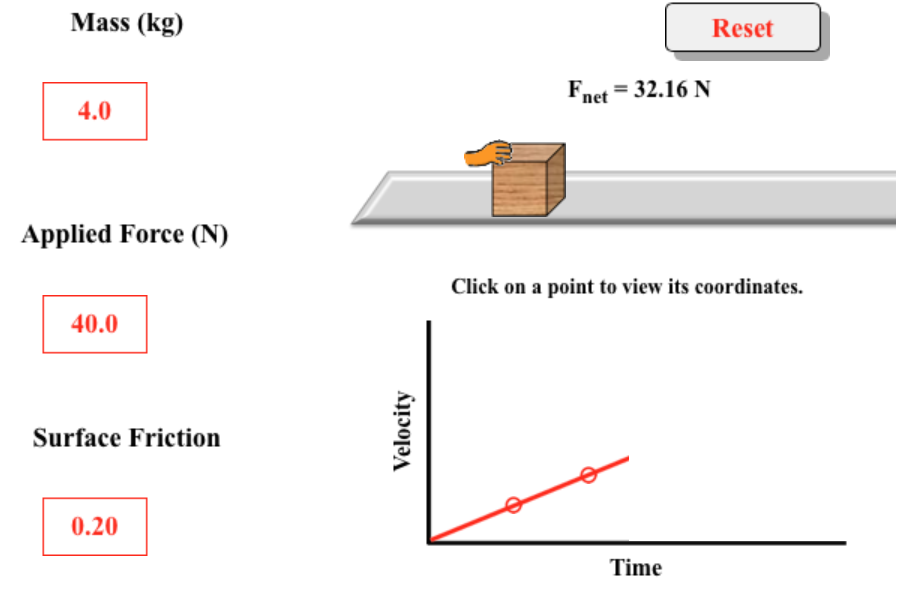###Push It!

Resource:

Force Interactive

Force Interactive - Exercise 1

Force Interactive - Exercise 2

Description:
This Interactive from The Physics Classroom blends a simulation and a classroom investigation to explore the effects of applied force on acceleration. The simulation provides a computationally accurate platform to conduct the studies. Students will decide what remains constant and which variables will change as they use the simulation to apply forces to a block moving along a one-dimensional path. As the simulation runs, a Velocity vs. Time graph is automatically generated.  Surface friction can be introduced to show how frictional force affects the motion. The accompanying student exercises provide guidance for investigating the relationships inherent in the simulation.

Performance Expectation:
Force and Motion  (HS-PS2-1):  Analyze data to support the claim that Newton’s Second Law of Motion describes the mathematical relationship among the net force on a macroscopic object, its mass, and its acceleration.

This activity aligns with the three dimensions of the Next Generation Science Standards in the manner described below:

Disciplinary Core Ideas
 Force and Motion (HS-PS2.A.1) Newton’s Second  Law accurately predicts changes in the motion of macroscopic objects. Students will use Newton’s Second Law to calculate net force and acceleration based upon data obtained from the simulation Force Interactive.  Through guided analysis of the data, they will draw conclusions based on evidence.

Crosscutting Concepts
 Cause and Effect – Grades 9-12:  Empirical evidence is required to differentiate between cause and correlation and make claims about specific causes and effects. In this activity, students will use a digital model of a block moving along a straight path (a simple physical situation appropriate for beginners). By manipulating different variables including mass, applied force to move the block, and friction, students will collect data and determine cause/effect relationships. The data collection will help learners understand that an applied force causes an acceleration in the direction of the force. The scientific question addressed are (1) What variables affect the acceleration and in what manner do they affect it? and (2) What is the effect of a varying applied force upon acceleration? Using data from their investigations, students will make claims about cause and effect.

Practices
 Analyzing and Interpreting Data – Grades 9-12:  Analyze data using computational models in order to make valid and reliable scientific claims. Taken together, the two activities in this Interactive explicitly address the practice of data analysis. To accurately interpret the data, students are called upon to generate equations expressing acceleration as a function of net force, mass, and applied force. For a more advanced investigation, friction can be introduced in the simulation and included in the data analysis. Engaging in Argument from Evidence – Grades 9-12:  Make and defend a claim based on evidence about the natural world that reflects scientific knowledge and student-generated evidence. The culminating task in both Exercises is the task of making an evidence-based scientific claim about the relationship of applied force and acceleration. To defend the claim, the student must write a paragraph explaining their own scientific reasoning, provide data from simulation-based trials, and generate graphs that show the linear regression statistics. Using Mathematics and Computational Thinking – Grades 9-12:  Use mathematical representations of phenomena to describe explanations. Students will use mathematics as they employ Newton’s Second Law to calculate net force, based upon data collected from the Force Interactive model. The first exercise prompts students to inspect collected data in order to determine how doubling, tripling, and quadrupling the mass and the net force will affect the acceleraton.

Associated Reading from The Physics Classroom

Other Supporting Pages at The Physics Classroom:

View Infographic.

### Search the NGSS Corner

Maybe you're looking for something really specific that pertains to a desired topic and emphasizes one or more of the listed NGSS dimensions. Why not try a search of this section of our website? Simply select from one or more of the pull-down menus and click Search.  This page will reload and a collection  of possibilities will be displayed in this section of the page and sorted by relevancy.